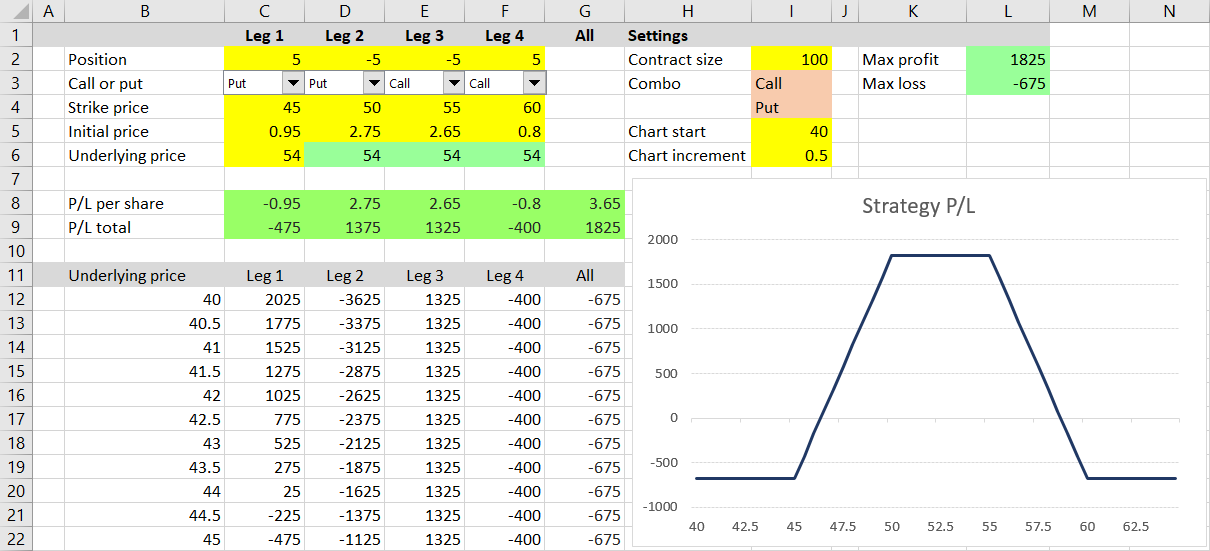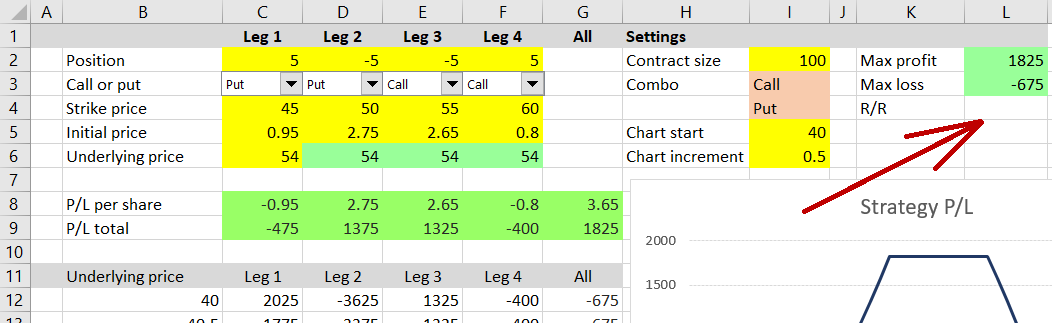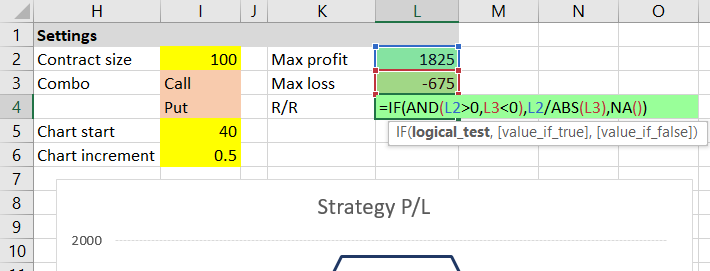# Calculating Option Strategy Risk-Reward Ratio

This is part 7 of the Option Payoff Excel Tutorial. In the previous part we have learned about useful properties of the payoff function and calculated maximum possible profit and maximum possible loss for an option strategy with up to four legs. In this part we will use the results to calculate another very useful statistic: the risk-reward ratio.## What Is Risk-Reward Ratio?

Risk-reward ratio, also known as reward-to-risk ratio or profit-loss ratio, is a measure that compares maximum possible profit we can gain from a trade with the risk (maximum possible loss) of the trade.

Its use is not limited to options. It is also widely used with futures, forex and many other kinds of trading, business, and speculation. It is often abbreviated as R/R or RRR.

## Format and Interpretation

Risk-reward ratio can be expressed in a number of different formats, most simply as:

maximum loss : maximum profit

In our example from the previous part, we have calculated maximum possible loss of \$675 and maximum potential profit of \$1,825. That would be a risk-reward ratio of:

675 : 1,825

In order to make the ratio easily comparable across different trades, it is common to divide both sides by maximum loss, which makes the left side always equal to one and the right side equal to maximum profit divided by maximum loss:

1 : maximum profit / maximum loss

In our example:

1 : 1,825 / 675

1 : 2.70

Sometimes the ratio is expressed as a single number – just the right side:

maximum profit / maximum loss

In our example, the risk-reward ratio in this format is 2.70. It would be more accurate to call this number "reward-to-risk" ratio.

That said, the exact format or how we call it is not that important, as long as we understand what our number means: It means how many multiples of the amount at risk we can possibly gain from the trade in ideal case. The higher the number, the better the trading opportunity, other things being equal.

## Calculating Risk-Reward Ratio in Excel

In our spreadsheet we will use the single number format and calculate risk-reward ratio in cell L4.As you have certainly guessed, the formula will divide maximum profit in cell L2 by maximum loss in cell L3:

``=L2/L3``

That said, loss in cell L3 is expressed as a negative number in our calculator, which would make the resulting risk-reward ratio also negative. Let's fix that by putting L3 inside an ABS function (absolute value):

``=L2/ABS(L3)``

## Infinite or Impossible Profit or Loss

We will make one more adjustment to handle special situations.

Firstly, for some option positions, maximum profit or maximum loss are infinite. In such cases risk-reward ratio can't be calculated (or it is infinitely big or infinitely small).

Secondly, there can be situations when all the possible outcomes from a trade are profitable (maximum possible loss is in fact still a profit) or all are losses (maximum possible profit is negative). These situations can happen when you enter different legs at different times, roll over parts of your position, or in some unlikely arbitrage situations. In such cases risk-reward ratio also doesn't make sense, because there is no risk or no real reward.

We will address both the above mentioned types of situations by adding an IF condition to our formula.

We will make the formula only return a number if the calculated maximum profit is a positive number (not negative and not infinite) and if the maximum loss is a negative number (not positive and not infinite).

Remember that in the previous part of the tutorial we have designed the maximum profit and maximum loss formulas in cells L2 and L3 in a way that returns "Infinite" (a word, not a number) for infinite values.

The formula for risk-reward ratio in cell L4 is:

``=IF(AND(L2>0,L3<0),L2/ABS(L3),NA())``It is an IF function which (as you already know) has three parts:

• The condition – in our case there are two conditions inside an AND function, which means both must be true (L2 must be a positive number and at the same time L3 must be a negative number)
• Result when condition is true – in our case the risk-reward ratio, calculated as `L2/ABS(L3)`
• Result when condition is false – in our case the NA function, which returns the standard Excel #N/A (not available) error

Note: As a challenge, you can expand the formula to return more specific messages when risk-reward ratio is not calculated (for example: "Infinite max profit", "Infinite max loss" or "No profit possible"), by replacing the NA() part with some more IF statements, or by changing the entire formula.

This is the end of this section. We have expanded our spreadsheet to also calculate risk-reward ratio of a given option strategy.

## Next Steps

We are approaching the end of the tutorial and there is not much left to calculate. One last thing, which can also be very useful when evaluating potential option trades, is the break-even point – the exact underlying price where P/L turns from loss to profit and vice versa. We will calculate break-even points (there can be more than one for some strategies) in the next section.

Go to next part: Calculating Option Strategy Break-Even Points

By remaining on this website or using its content, you confirm that you have read and agree with the Terms of Use Agreement.

We are not liable for any damages resulting from using this website. Any information may be inaccurate or incomplete. See full Limitation of Liability.

Content may include affiliate links, which means we may earn commission if you buy on the linked website. See full Affiliate and Referral Disclosure.

We use cookies and similar technology to improve user experience and analyze traffic. See full Cookie Policy.